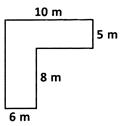Courses

# Test: Mensuration - 3

## 20 Questions MCQ Test Mathematics (Maths) Class 6 | Test: Mensuration - 3

Description
This mock test of Test: Mensuration - 3 for Class 6 helps you for every Class 6 entrance exam. This contains 20 Multiple Choice Questions for Class 6 Test: Mensuration - 3 (mcq) to study with solutions a complete question bank. The solved questions answers in this Test: Mensuration - 3 quiz give you a good mix of easy questions and tough questions. Class 6 students definitely take this Test: Mensuration - 3 exercise for a better result in the exam. You can find other Test: Mensuration - 3 extra questions, long questions & short questions for Class 6 on EduRev as well by searching above.
QUESTION: 1

Solution:
QUESTION: 2

Solution:
QUESTION: 3

### What is the perimeter of an equilateral triangle of side a units?

Solution:
QUESTION: 4

What do you call the total boundary length of a closed figure?

Solution:
QUESTION: 5

What is the amount of surface enclosed by a closed figure called?

Solution:
QUESTION: 6

The perimeter of a rectangle is 170 m and its length is 50 m. What is its breadth?

Solution:
QUESTION: 7

The area of a rectangle is 630 sq. cm and its breadth is 15 cm. What is its length?

Solution:

length x  breadth = 630

length = 630/breadth

= 630/15

length = 42 cm

QUESTION: 8

Samuel wanted to erect some vertical stones along the boundary of his plot at a distance of 10 m each. If the length of the plot is 30 m and the breadth is 15 m how many stones are required?

Solution:

Perimeter of plot = 2(l+b) = 2(30+15) = 90m

Distance between two stones = 10m

Therefore, number of stones = 90/10 = 9

QUESTION: 9

The length and breadth of a rectangle are 3.2 m and 150 cm. What is its area?

Solution:

We know that,

Area=l∗b

l=3.2m
b=1.5m
l×b=3.2×1.5 =4.80 sq m

QUESTION: 10

80 students of the same height stand with both hands stretched all along the sides of a rectangular garden, each student covering a length of 1.75 m. What is the perimeter of the garden?

Solution:

80 × 1.75 m = 140 m

QUESTION: 11

A table top is covered with 25 squares of equal size. The side of the square is 3 cm. What is the area of the table top?

Solution:

Area of square
= s × s
= 3×3 = 9 sq. cm
Area of 25 squares
= 25 × 9
= 225 sq.cm

QUESTION: 12

The length and breadth of a rectangular plot are 900 m and 700 m respectively. If three rounds offence is fixed around the field at the cost of Rs. 8 per metre, what is the total amount spent?

Solution:

l = 900 m, b = 700 m
Perimeter = 2 (900 + 700) m = 2(1600) m = 3200 m
Perimeter for 3 rounds fence = 3(3200) m = 9600 m
∴  Total amount spent
= 9600×Rs. 8 = Rs. 76,800

QUESTION: 13

How many sq. cm make a sq. m?

Solution:

1 sq. m = 10,000 sq. cm

QUESTION: 14

A wooden plank measures 6 m in length and 3 m in breadth. If five such wooden planks are arranged in order, what is the area occupied by them?

Solution:

l = 6m; b = 3m
Area of one plank = 6×3 = 18sq.m
Number of wooden planks = 5
Area of 5 wooden planks = 18×5 = 90 m2

QUESTION: 15

On a wall of dimensions 10.5 m long and 8.5 m wide, a square shaped wall poster is stuck at the centre whose side measure is 2.5 m. If the remaining part of the wall is to be painted with pink colour costing Rs. 12 per sq. m, how much does it cost?

Solution:

Area of the poster = 2.5 × 2.5 sq. m
= 6.25sq.m Area of the wall
= 10.5×8.5 sq.m
= 89.25sq.m
Area of the wall to be painted =(89.25-6.25) sq.m = 83.00 sq.m
Cost of painting = 83 × Rs. 12 = Rs. 996

QUESTION: 16

What is the area of the given figure?Solution:

10×5 = 50sq.m
6×8 = 48 sq. m
∴ The required area = 50 + 48 = 98 sq. m

QUESTION: 17

In a square shaped park, whose side measures 28 m, a rectangular pond is located at the centre with dimensions 3 m and 2 m. What is the area of the park excluding the pond?

Solution:

Area of pond = 3m × 2m = 6 sq m
Area of park = 28 × 28
= 784 sq m
Area of the park excluding the pond
= 784-6
= 778 sq m

QUESTION: 18

An isosceles triangle has a measure of p units for its equal sides and q units for its unequal side. What is its perimeter?

Solution:
QUESTION: 19

What is the resultant of twice the sum of the length and breadth of a rectangle?

Solution:
QUESTION: 20

The perimeter of a square is 728 cm. Find the measure of its side.

Solution: